Courses

# Direct Current Machine Of Electrical Machines

## 10 Questions MCQ Test Topicwise Question Bank for GATE Electrical Engineering | Direct Current Machine Of Electrical Machines

Description
This mock test of Direct Current Machine Of Electrical Machines for Electrical Engineering (EE) helps you for every Electrical Engineering (EE) entrance exam. This contains 10 Multiple Choice Questions for Electrical Engineering (EE) Direct Current Machine Of Electrical Machines (mcq) to study with solutions a complete question bank. The solved questions answers in this Direct Current Machine Of Electrical Machines quiz give you a good mix of easy questions and tough questions. Electrical Engineering (EE) students definitely take this Direct Current Machine Of Electrical Machines exercise for a better result in the exam. You can find other Direct Current Machine Of Electrical Machines extra questions, long questions & short questions for Electrical Engineering (EE) on EduRev as well by searching above.
QUESTION: 1

### Consider the following statements about the field windings used for various types of dc machines: 1. For dc shunt machines, large number of turns of small cross-section are used. 2. For dc series machine, small number of turns of large cross-section are used. 3. For dc compound machine, both shunt (thick wire) and series (thin wire) field windings are used. Out of the above statements the correct statements is/are:

Solution:

For dc shunt machine, field current is very less, therefore for required field AT/Pole, number of turns of field winding is increased. Thus, R should be high for low If.
For dc series machine field winding current is equal to armature current which is of larger value. Therefore, number of turns of field winding is reduced to get the required field AT/Pole. As it has to handle large current, therefore resistance of field winding should be less by keeping the winding thick. Statement-3 is wrong.

QUESTION: 2

### A 6-pole dc machine has 300 conductors and flux per pole is 15 mWb, If the machine Is driven at 1800 rpm, then the average emf induced per conductor will be

Solution:

Given, speed,
N= 1800 rpm
n = 1800/60 rps = 30 rps
Average emf induced per conductor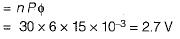QUESTION: 3

### A commutator with a diameter of 50 cm rotates at 1000 rpm. For a brush width of 1.5 cm, the time of commutation is

Solution:

Peripheral velocity of commutator is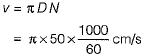Now, brush width = v x time of commutation

or, time of commutation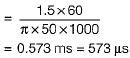QUESTION: 4

A 24 kW, 250 V, 1600 rpm separately-excited d.c. generator has armature circuit resistance of 0.1Ω. The machine is first run at rated speed and the field winding current is adjusted to give an open circuit voltage of 260 V. Now, when the generator is loaded to deliver its rated current, the speed of the driving motor is found to be 1500 r.p.m. Assuming flux to remain constant, the terminal voltage of the generator under these conditions is

Solution:

We know that,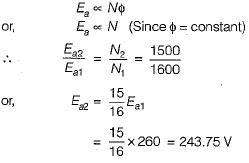Now, rated armature current is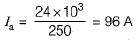So, the terminal voltage is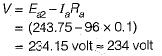QUESTION: 5

Assertion (A): DC series motors are suitable for buses, trolleys, hoists and trains.
Reason (R): DC series motors are variable Speed motors.

Solution:

The reason of assertion is that dc series motor develop high torque at start due to which these motors are used for hard to start loads. Reason is also correct because these are variable speed motors.

QUESTION: 6

Assertion (A): Armature resistance control method of speed control of dc motors is wasteful and inefficient.
Reason (R): Large amount of power is wasted in the control resistances, specially in case of shunt motors'.

Solution:
QUESTION: 7

Match List-I (Tests on DC machines) with
List-ll (Results) and select the correct answer using the codes given below the lists:
List-I
A. Field test
B. Swinburne’s test
C. Hopkinson test
D. Retardation test
List-II
1. Stray losses in case of dc series machines
2. Constant losses
3. Performance of machines regarding commutation and temperature rise
4. Stray losses in case of dc shunt machines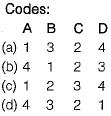Solution:
QUESTION: 8

At a certain speed and flux, the voltage generated by a dc generator is 230 volts. If the speed is increased by 20% and the flux is simultaneously reduced by 10%, the voltage generated will be

Solution:

We know that,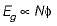∴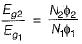or,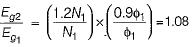∴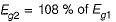Thus, voltage generated is increased by 8%.

QUESTION: 9

LAP winding is employed in a dc machine of

Solution:

For LAP winding, number of parallel path = number of poles.
Thus, resistance is less compared to wave winding. Hence, current rating is more and voltage rating is less compared to wave winding.

QUESTION: 10

Consider the following parts of a dc machine:
1. Pole core
2. Brushes
3. Yoke
4. Armature core
Which of the above parts are not subjected to iron loss?

Solution: